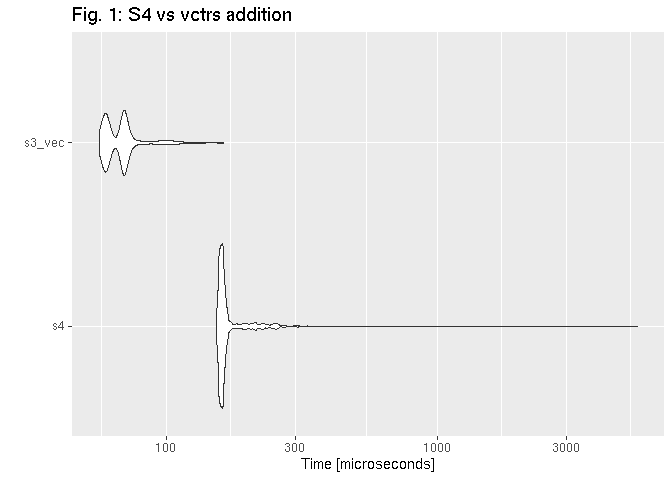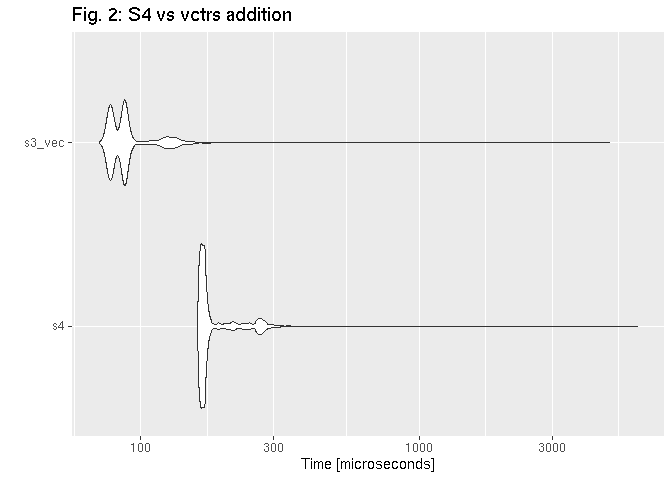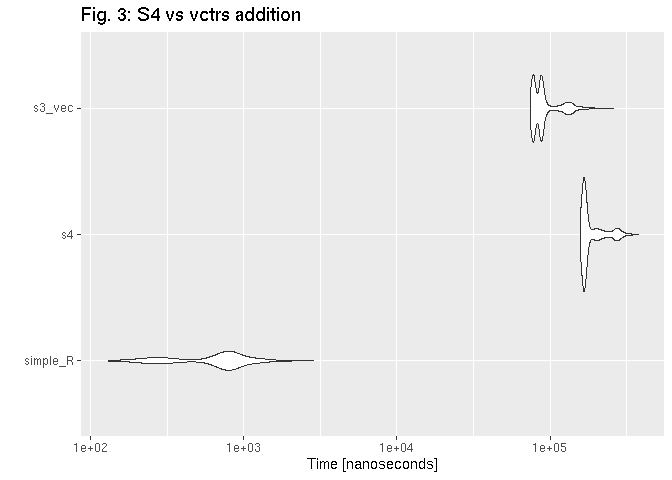Want to share your content on R-bloggers? click here if you have a blog, or here if you don't.## Why do we may need double dispatch?

In most cases, when writing R scripts or even creating R packages, it is
enough to use standard functions or S3 methods. However, there is one
important field that forces us to consider double dispatch question:
arithmetic operators.

Suppose we’d like to create a class, which fits the problem we’re
currently working on. Let’s name such class beer.

```beer <- function(type){
structure(list(type  = type),class = "beer")
}

opener <- function(){
structure(list(), class = "opener")
}

pilsner <- beer("pilnser")
my_opener <- opener()
```

Then, we create an operator which defines some non-standard behaviour.

• if we add an opener to the beer, we get an opened_beer.
• adding a numeric x, we get a case of beers (which even contain
a negative number of bees, i.e. our owe…)
• if second argument is different than a or opener or numeric,
we get… untouched beer

Let’s demonstrate, how does it work:

````+.beer` <- function(a, b){
if (inherits(b, "opener")) {
return(structure(list(
name  = paste("opened", a\$name)
), class = "opened_beer"))
} else if (inherits(b, "numeric")) {
print("It's magic! You've got a case of beers!")
return(structure(list(
n_beers = 1 + b
), class = "case_of_beers"))
} else {
return(a)
}
}

pilsner + my_opener

## \$name
##  "opened "
##
## attr(,"class")
##  "opened_beer"

pilsner + -0.1

##  "It's magic! You've got a case of beers!"

## \$n_beers
##  0.9
##
## attr(,"class")
##  "case_of_beers"
```

Don’t you think, that such operations should be commutative?

```my_opener + pilsner

## list()
## attr(,"class")
##  "opener"
```

What did happen here? This is an example of the way the R interpreter
handles arithmetic operator. It was described with details on Hiroaki
Yutani’s
blog
.
Briefly speaking, in this particular case R engine matched method to the
second argument (not to the first one), because there is no `+.opener`
S3 method. What about such trick:

````+.opener` <- function(a, b) b + a
```

After that, the result is different:

```my_opener + pilsner

## Warning: Incompatible methods ("+.opener", "+.beer") for "+"

## Error in my_opener + pilsner: non-numeric argument to binary operator
```

We crashed our function call. When both objects have the `+` method
defined and these methods are not the same, R is trying to resolve the
conflict by applying an internal `+`. It obviously cannot work. This
case could be easily solved using more ‘ifs’ in the `+.beer` beer
function body. But let’s face a different situation.

```-0.1 + pilsner

##  -0.1
```

What a mess! Simple S3 methods are definitely not the best solution when
we need the double dispatch.

## S4 class: a classic approach

To civilize such code, we can use classic R approach, S4 methods. We’ll
start from S4 classes declaration.

```.S4_beer          <- setClass("S4_beer", representation(type = "character"))
.S4_opened_beer   <- setClass("S4_opened_beer", representation(type = "character"))
.S4_opener        <- setClass("S4_opener", representation(ID = "numeric"))
.S4_case_of_beers <- setClass("S4_case_of_beers", representation(n_beers = "numeric"))
```

Then, we can two otptions, how to handle `+` operators. I didn’t mention
about it in the previous example, but both S3 and S4 operators are
S3,
S4).

We can set a S4 method for a single operator and that looks as follows:

```setMethod("+", c(e1 = "S4_beer", e2 = "S4_opener"),
function(e1, e2){
if (inherits(e2, "S4_opener")) {
return(.S4_opened_beer(type  = paste("opened", [email protected])))
} else if (inherits(e2, "numeric")) {
print("It's magic! You've got a case of beers!")
return(.S4_case_of_beers(n_beers = 1 + e2))
} else {
return(e1)
}
})

setMethod("+", c(e1 = "S4_opener", e2 = "S4_beer"),
function(e1, e2) e2 + e1)
```

Alternatively, we can define a method for `Arith` geneneric and check,
what method is exactly called at the moment. I decided to use the second
approach, because it’s more similar to the way the double dispatch is
implemented in the vctrs library.

```.S4_fun <- function(e1, e2){
if (inherits(e2, "S4_opener")) {
return(.S4_opened_beer(type  = paste("opened", [email protected])))
} else if (inherits(e2, "numeric")) {
print("It's magic! You've got a case of beers!")
return(.S4_case_of_beers(n_beers = 1 + e2))
} else {
return(e1)
}
}

setMethod("Arith", c(e1 = "S4_beer", e2 = "S4_opener"),
function(e1, e2)
{
op = .Generic[]
switch(op,
`+`  = .S4_fun(e1, e2),
stop("undefined operation")
)
})

setMethod("Arith", c(e1="S4_opener", e2="S4_beer"),
function(e1, e2)
{
op = .Generic[]
switch(op,
`+`  = e2 + e1,
stop("undefined operation")
)
})
```

Let’s create our class instances and do a piece of math.

```S4_pilsner <- .S4_beer(type = "Pilsner")
S4_opener <- .S4_opener(ID = 1)

S4_pilsner + S4_opener

## An object of class "S4_opened_beer"
## Slot "type":
##  "opened Pilsner"

S4_opener + S4_pilsner

## An object of class "S4_opened_beer"
## Slot "type":
##  "opened Pilsner"
```

Declared methods are clear, and, the most important: they work
correctly.

## vctrs library: a tidyverse approach

vctrs is an interesting library,
thought as a remedy for a couple of R disadvantages. It delivers, among
others, a custom double-dispatch system based on well-known S3
mechanism.

At the first step we declare class ‘constructors’.

```library(vctrs)

.vec_beer <- function(type){
new_vctr(.data = list(type  = type), class = "vec_beer")
}

.vec_opened_beer <- function(type){
new_vctr(.data = list(type  = type), class = "vec_opened_beer")
}

.vec_case_of_beers <- function(n_beers){
new_vctr(.data = list(n_beers  = n_beers), class = "vec_case_of_beers")
}

.vec_opener <- function(){
new_vctr(.data = list(), class = "vec_opener")
}
```

Then, we create class instances.

```vec_pilsner   <- .vec_beer("pilnser")
vec_opener <- .vec_opener()
print(class(vec_pilsner))

##  "vec_beer"   "vctrs_vctr"

print(class(vec_opener))

##  "vec_opener" "vctrs_vctr"
```

At the end, we write a double-dispatched methods in vctrs style. As
you can see,

```.fun <- function(a, b){
if (inherits(b, "vec_opener")) {
return(.vec_opened_beer(type  = paste("opened", a\$type)))
} else if (inherits(b, "numeric")) {
print("It's magic! You've got a case of beers!")
return(.vec_case_of_beers(n_beers = 1 + b))
} else {
return(a)
}
}

vec_arith.vec_beer <- function(op, x, y, ...) {
UseMethod("vec_arith.vec_beer", y)
}

vec_arith.vec_opener <- function(op, x, y, ...) {
UseMethod("vec_arith.vec_opener", y)
}

vec_arith.vec_beer.vec_opener <- function(op, x, y, ...){
switch(op,
`+` = .fun(x, y),
stop_incompatible_op(op, x, y)
)
}

vec_arith.vec_opener.vec_beer <- function(op, x, y, ...){
y + x
}

vec_pilsner + vec_opener

##
##           type
## opened pilnser

vec_opener + vec_pilsner

##
##           type
## opened pilnser
```

It works properly, too.

## Benchmark

I’ve created all the classes and methods above not only to demonstate,
how to implement double dispatch in R. My main goal is to benchmark both
approaches and check, which one has smaller overhead. The hardware I
used for the test looks as follows:

```## \$vendor_id
##  "GenuineIntel"
##
## \$model_name
##  "Intel(R) Core(TM) i3 CPU       M 350  @ 2.27GHz"
##
## \$no_of_cores
##  4

## 8.19 GB

sessionInfo()

## R version 3.6.1 (2019-07-05)
## Platform: x86_64-pc-linux-gnu (64-bit)
## Running under: Ubuntu 18.04.2 LTS
##
## Matrix products: default
## BLAS:   /usr/local/lib/R/lib/libRblas.so
## LAPACK: /usr/local/lib/R/lib/libRlapack.so
##
## locale:
##   LC_CTYPE=pl_PL.UTF-8       LC_NUMERIC=C
##   LC_TIME=pl_PL.UTF-8        LC_COLLATE=pl_PL.UTF-8
##   LC_MONETARY=pl_PL.UTF-8    LC_MESSAGES=en_US.utf8
##   LC_PAPER=pl_PL.UTF-8       LC_NAME=C
##  LC_MEASUREMENT=pl_PL.UTF-8 LC_IDENTIFICATION=C
##
## attached base packages:
##  stats     graphics  grDevices utils     datasets  methods   base
##
## other attached packages:
##  vctrs_0.2.3
##
## loaded via a namespace (and not attached):
##   Rcpp_1.0.3            benchmarkmeData_1.0.3 knitr_1.23
##   magrittr_1.5          tidyselect_0.2.5      doParallel_1.0.15
##   lattice_0.20-38       R6_2.4.0              rlang_0.4.2
##  foreach_1.4.7         httr_1.4.1            stringr_1.4.0
##  dplyr_0.8.3           tools_3.6.1           parallel_3.6.1
##  grid_3.6.1            xfun_0.9              htmltools_0.3.6
##  iterators_1.0.12      yaml_2.2.0            digest_0.6.25
##  assertthat_0.2.1      tibble_2.1.3          benchmarkme_1.0.3
##  crayon_1.3.4          Matrix_1.2-17         purrr_0.3.3
##  codetools_0.2-16      glue_1.3.1            evaluate_0.14
##  rmarkdown_1.14        stringi_1.4.3         pillar_1.4.2
##  compiler_3.6.1        pkgconfig_2.0.2
```

It’s my good old notebook, which is not a beast.

```library(microbenchmark)
library(ggplot2)
```

### Beer + opener

```bm1 <- microbenchmark(
s4 = S4_pilsner + S4_opener,
s3_vec = vec_pilsner + vec_opener,
times = 1000
)

## Unit: microseconds
##    expr     min       lq      mean   median       uq      max neval
##      s4 153.292 158.2120 178.40541 161.4225 165.6375 5506.681  1000
##  s3_vec  56.686  60.1265  69.52364  68.9240  70.8830  163.278  1000
```### Opener + beer

```bm2 <- microbenchmark(
s4 = S4_opener + S4_pilsner,
s3_vec = vec_opener + vec_pilsner,
times = 1000
)

## Unit: microseconds
##    expr     min       lq      mean   median       uq      max neval
##      s4 159.512 164.6735 191.74781 168.9655 176.3165 6068.477  1000
##  s3_vec  71.110  78.5835  96.22535  86.6720  89.4015 4796.377  1000
```### Bonus: opener + beer vs addtion of numerics

```bm3 <- microbenchmark(
simple_R = 1 + 2,
s4 = S4_opener + S4_pilsner,
s3_vec = vec_opener + vec_pilsner,
times = 1000
)

## Unit: nanoseconds
##      expr    min       lq      mean   median     uq    max neval
##  simple_R    130    344.0    697.49    744.5    857   2862  1000
##        s4 158769 164522.5 189297.35 169270.5 198120 375648  1000
##    s3_vec  74775  78395.5  94786.28  87192.5  94085 258129  1000
```## Conclusions

It seems that vctrs-based performs better than traditional S4
methods
. Obviously, I checked only one operation and probably some
edge cases may exists. However, I think that it shows us some direction,
what execution time we can expect.

## Further sources

If you are interesting, how to implement double-dispatched operators in
S4, I encourage you to get familiar with code of the following R
libraries:

If you are looking for some examples of vctrs, I recommend you to
learn the source code of: